PHY294H - Lecture 17

Capacitor/Resistor networks

Kirchhoff's laws still apply and are the basis for analysis of RC circuits. In analysing these circuits we need to relate the charge on the capacitors to the current flow into the capacitor. The relationship we need is simply i = dQ/dt. This is combination with Kirchhoff's laws enable us to solve RC circuits. We shall be interested in the way that capacitors in a circuit charge and discharge. We shall also be interested in the long time behavior (steady state) in which each capacitor has reach a final charge state. To study charging, we will close a switch in the circuit and will analyse what happens as charge either flows onto or off of the capacitors.

A resistor and capacitor in series

Consider Kirchhoff's loop law for a capacitor, C, resistor R and an emf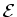in series. At long times, this circuit carries no current and the emf is dropped across the capacitor. The circuit is only interesting if we have a switch in the circuit which we can open and close as we wish. Analysis of the time dependence of the current in the circuit and the charge across the capacitor is a study of transients.

Charging a capacitor

Consider placing a switch in the circuit and the situation in which the capacitor initially has charge Q(t=0) = 0. We close the switch at t=0 and the capacitor begins to charge.(1)

In order to proceed we use the relation between current and charge, ie. i = dQ/dt. From this we find,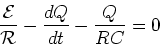(2)

In the steady state, i = dQ/dt=0 and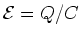. The time dependence of the charging of the capacitor is given by the solution to Eq. (2), which is,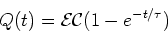(3)

where the time constant. Note that Q(0) = 0 and that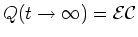. The current as a function of time is given by,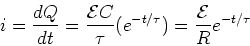(4)

Discharging a capacitor

Now consider a capacitor which has charge Q0 in a circuit with a resistor and an open switch. The switch is closed at t=0. Find the current in the circuit and the charge on the capacitor as a function of time. Kirchhoff's loop law gives,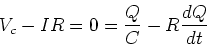(5)

The solution to this equation is,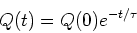(6)

The current in the circuit is,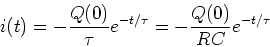(7)

Energy storage and dissipation in an RC circuit

In the charging process above, the energy supplied by the emf (battery) is(8)

half of this energy is stored in the capacitor and half of this energy is dissipated in the resistor.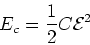(9)

The energy dissipated in the resistor is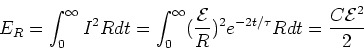(10)

FGT 28.59

Consider an emfin parallel with a resistor R2 and in parallel with a series combination of a resistor R1 and a capacitor C. A switch is placed near the emf. At times t<0 the capacitor is uncharged and the switch is open. At t=0 the switch is closed. Find an expression for the current through the battery, I(t), as a function of time and the charge on the capacitor Q1(t), also as a function of time.

Kirchhoff's junction law gives,

 I = I1 + I2 (11)

where I1 and I2 are the currents through resistors R1 and R2 respectively. Kirchhoff's loop law gives,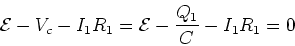(12)

and,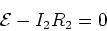(13)

For times t<0, there is no current in the circuit as the switch is open. At time t=0 the switch is closed. At that time, there is no charge on the capacitor, so there is no voltage across the capacitor. Therefore the circuit acts like a parallel combination of resistors R1 and R2. We thus have,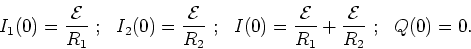(14)

At long times, the capacitor is fully charged and we have,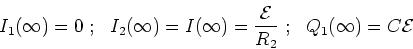(15)

To find the time dependence of the current I1 we use I1 = dQ1/dtin Eq. (12). This yields,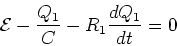(16)

This has the solution,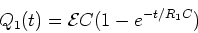(17)

The current I1(t) is then,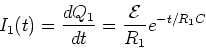(18)

Using Kirchhoff's junction rule, we then find,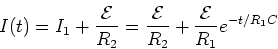(19)